Courses

# Introduction to Rigid Body Motion Civil Engineering (CE) Notes | EduRev

## Civil Engineering (CE) : Introduction to Rigid Body Motion Civil Engineering (CE) Notes | EduRev

The document Introduction to Rigid Body Motion Civil Engineering (CE) Notes | EduRev is a part of the Civil Engineering (CE) Course Introduction to Dynamics and Vibrations- Notes, Videos, MCQs.
All you need of Civil Engineering (CE) at this link: Civil Engineering (CE)

6.1 Introduction

In practice, it is often not possible to idealize a system as a particle. In this section, we construct a more sophisticated description of the world, in which objects rotate, in addition to translating. This general branch of physics is called ‘Rigid Body Dynamics.’

Rigid body dynamics has many applications. In vehicle dynamics, we are often more worried about controlling the orientation of our vehicle than its path – an aircraft must keep its shiny side up, and we don’t want a spacecraft tumbling uncontrollably. Rigid body mechanics is used extensively to design power generation and transmission systems, from jet engines, to the internal combustion engine, to gearboxes. A typical problem is to convert rotational motion to linear motion, and vice-versa. Rigid body motion is also of great interest to people who design prosthetic devices, implants, or coach athletes: here, the goal is to understand human motion, to protect athletes from injury or improve their performance, or to design devices that replicate the complicated motion of a human joint correctly. For example, Professor Crisco’s orthopaedics lab at Brown studies human motion and the forces they generate at human joints, to help understand how injuries occur and how they can be prevented.

The motion of a rigid body is often very counter-intuitive. That’s why there are so many toys that exploit the properties of rigid bodies: the motion of a spinning top; a boomerang; the ‘rattleback’ and a Frisbee can all be explained using the equations derived in this section.

Here is a quick outline of how we analyze motion of rigid bodies.

1. A rigid body is idealized as an infinite number of very small particles.

2. We already know the equations of motion for a system of particles (Section 4 of the notes):

The force-momentum equation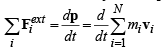The moment – angular momentum equation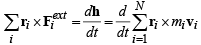The work-kinetic energy equation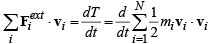3. These equations tell us how a rigid body moves. But to use them, we would need to keep track track of an infinite number of particles! To simplify the problem, we set up some mathematical methods that allow us to express the position and velocity of every point in a rigid body in terms of the position rG , velocity vG and acceleration aG of its center of mass, and its rotation tensor R(quantifying its orientation) and its angular velocity ω , and angular acceleration α . This allows us to write the linear momentum, angular momentum, and kinetic energy of a rigid body in the form

p = MvG  h =rG × MvG+ IGω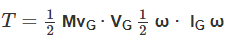where M is the total mass of the body and IG is its mass moment of inertia.

4. We can then derive the rigid body equations of motion: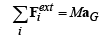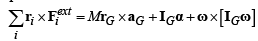Offer running on EduRev: Apply code STAYHOME200 to get INR 200 off on our premium plan EduRev Infinity!

## Introduction to Dynamics and Vibrations- Notes, Videos, MCQs

20 videos|53 docs

,

,

,

,

,

,

,

,

,

,

,

,

,

,

,

,

,

,

,

,

,

;